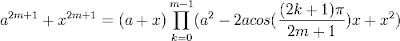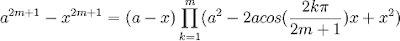## Friday, December 21, 2007

### Roger Cotes' Formulas

Roger Cotes thoroughly investigated the factorization of x4 + a4. In doing so, he found the following formulas which he presented without proof:

Formula 1:Formula 2:Formula 3:Formula 4:These formulas demonstrated that Leibniz's supposition about x4 + a4 was incorrect.

The proof for these formulas would later be provided by Abraham de Moivre as he investigated the properties of the roots of unity.

References: